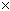SEARCH HOMEMath Central Quandaries & QueriesQuestion from Paul: I have an above ground pool that is 585 sq feet. I want to cover the bottom in 3 inches of sand. The sand supplier says I need  about 6 cubic yards, based on your prior answers on this subject. I come up with 1.8 yards. What  is  the  correct amount? ThanksPaul,

The volume you are trying to fill has an area of 585 square feet (that's 65 square yards) and a depth of 3 inches (that's 1/12 of a yard). Thus the volume is 65/12 = 5.4 cubic yards of sand.

I'm not sure how you arrived at 1.8 yards. Try it again using our "prior answers" and if you still get the wrong result, email us about the answer you are referencing so we can check it.

You can also calculate volumes using our volume calculator page.

Stephen La Rocque. >

Paul emailed us back:

i ARRIVED AT 1.8 YARDS USING THE ANSWER TO BETH, I believe that the fraction used was wrong, 1/108.

Thanks
Paul

Paul, I think you are referring to this web page, right? The difference between your answer and the correct answer is a factor of 1/3. This is likely because on Beth's question I explicity converted from inches to yards by multiplying her depth in inches by 1 foot/12 inches and then by 1 yard/3 feet . So you saw a factor of 1/12 and a factor of 1/3 in dealing with the depth.

In your question, I just said 3 inches is 1/12 of a yard. This is a coincidental match to the factor in Beth's answer which is easy to confuse. If we use the method in her problem to solve your problem then yours would be 3 inches1 ft/12 in1 yd/3 ft and we would still get 1/12 of a yard.

Stephen La Rocque.>Math Central is supported by the University of Regina and The Pacific Institute for the Mathematical Sciences.